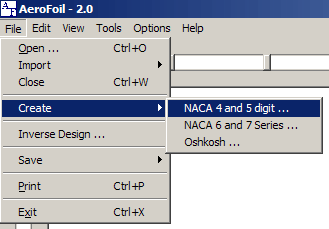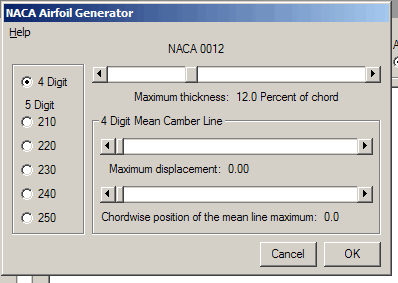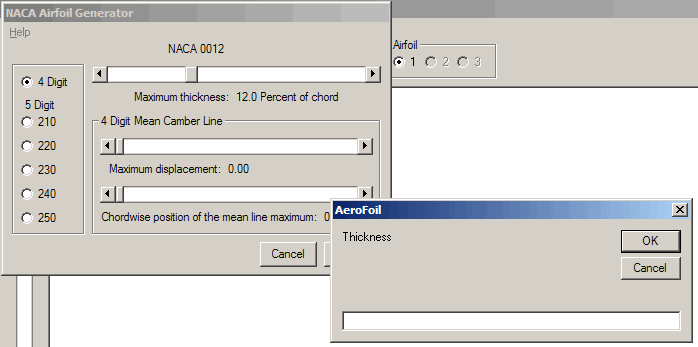## NACA 4 and 5 digit AirfoilsNACA 4 and 5 digit wing sections combine a thickness distribution with a camber line. In the early 30’s, NACA found that the successful airfoils of the time had very similar thickness distributions after their camber lines were straightened. The available data was used to create an equation for a thickness distribution that was similar in turn to those airfoils. That equation is:

y = +/- t (0.29690 x0.5 - 0.12600 x - 0.35160 x2 + 0.28430 x3 - 0.10150 x4) / 0.20

where t is the thickness expressed as a fraction of the chord.

The camber line for a 4-digit airfoil is given by:
yc = m (2 p x - x2) / p2 forward of the maximum ordinate, and
yc = m [(1-2 p) + 2 p x - x2] / (1 - p)2 aft of the maximum ordinate

where m is the maximum ordinate of the mean line expressed as a fraction of the chord and p is the chordwise location of the maximum ordinate.

The camber line for a 5-digit airfoil is given by:
yc = k1 [x3 - 3 m x2 + m2 (3 - m) x] / 6 from x = 0 to x = m, and
yc = k1 m3 (1 - x) from x = m to x = 1

Values of m were chosen to give five positions of p, the maximum camber, of 0.05c, 0.10c, 0.15c, 0.20c, and 0.25c, where c in the airfoil chord. Values of k1 were calculated to result in a design lift coefficient of 0.3.

 Mean Line Designation p m k1 210 0.05 0.0580 361.4 220 0.10 0.1260 51.64 230 0.15 0.2025 15.957 240 0.20 0.2900 6.643 250 0.25 0.3910 3.230

The mean line and thickness distribution are combined to give the airfoil shape by the following:
xu = x – y Sin θ
yu = yc + y Cos θ
xl = x + y Sin θ
yl = yc - y Cos θ
where xu and yu are the upper surface coordinates, xl and yl are the lower surface coordinates, and θ is the angle between the mean line and the airfoil chord.

Various modification of the mean line, leading edge radius of curvature, and trailing edge angle were made as a part of the NACA research; however, these are not implemented in AeroFoil.

The slider bars are used to change the thickness and camber line parameters. Camber line slider bars are only used for the four digit airfoils, five digit airfoil camber lines are the NACA default values. Values used to define the thickness and camber line may also be entered manually. Left click over the numeric value and a text box will open, type the desired value, close the window, and the value will be updated.